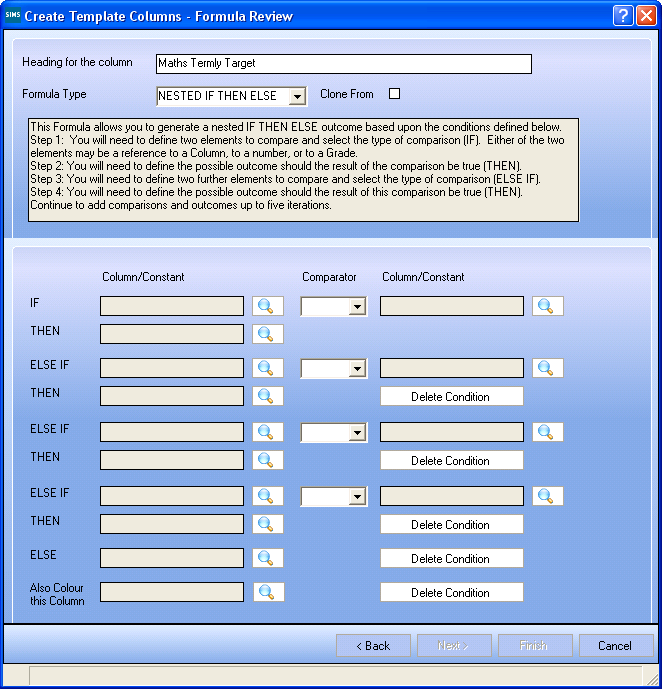# Adding a Cloned Formula Column for Data Review

 Page 63/209 Date 08.07.2018 Size 12.14 Mb.

#### Adding a Cloned Formula Column for Data Review

Formula columns can be cloned to reduce the amount of time it takes to create a template. This would be particularly useful if you wish to use an existing formula column as the framework for one or more new ones, meaning you do not have to create a brand new formula from scratch. When a formula column is cloned, it is automatically saved and can itself be used to clone from in future.

NOTE: Only IF THEN ELSE and NESTED IF THEN ELSE formulae can be cloned. These formulae can be cloned only if the selected template already contains an IF THEN ELSE or a NESTED IF THEN ELSE formula.

Only formulae that already exist in the selected template can be cloned. It is not possible to clone formulae from other templates.

1. Follow steps 1 to 3 in Adding Formula Columns for Data Review to select the required template.

2. Select either IF THEN ELSE or NESTED IF THEN ELSE from the Formula Type drop-down list.If the template already contains a formula column of the selected type, the